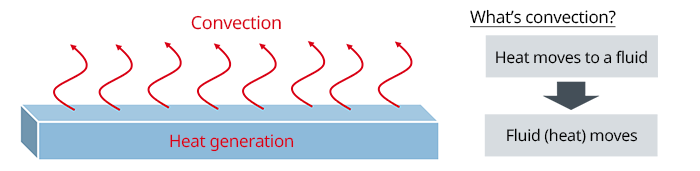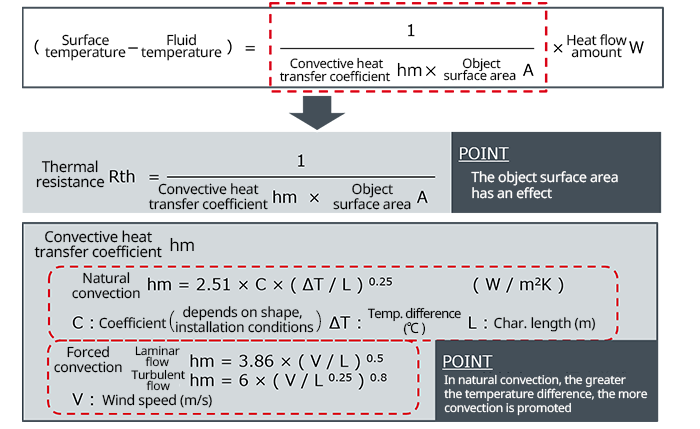# Fundamentals of Thermal Resistance and Heat Dissipation : Thermal Resistance in Convection

2021.04.21

・A fluid is something that flows, whether a gas, a liquid, or the like.

・Convection is a phenomenon of heat movement in which a fluid that has received heat moves, thereby transferring heat.

・Natural convection is flow driven by the buoyancy that arises from temperature differences in the fluid.

・Forced convection is flow driven by external factors, such as a fan or a pump.

・Thermal resistance in convection is the reciprocal of the product of the convective heat transfer coefficient hm and the surface area A of the object emitting heat.

In succession to the previous article on thermal resistance in heat conduction, this article discusses thermal resistance in convection.

There are a number of kinds of convection; below we provide definitions, along with terminology. The diagram is a conceptual image of convection.

Fluids Something that flows, such as gases and liquids A movement phenomenon in which heat is transported by movement of a fluid that has received heat*Where no fluid is present (vacuum), heat movement by convection cannot be expected to occur Flow is driven solely by buoyancy due to temperature differences in a fluid Flow is driven by an external factor, such as a fan or pump## Thermal Resistance in Convection

The following equations represent thermal resistance in convection.Thermal resistance in convection is the reciprocal of the product of the convective heat transfer coefficient hm and the surface area A of the object emitting heat. From the equation, we see that as the surface area of the object increases, the thermal resistance of convection decreases.

The convective heat transfer coefficient hm differs depending on the type of convection. We have presented hm values for both natural convection and for forced convection (laminar and turbulent flow).